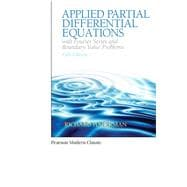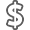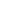Applied Partial Differential Equations with Fourier Series and Boundary Value Problems (Classic Version)

• ISBN13:

• ISBN10:

0134995430

• Edition: 5th
• Format: Paperback
• Publisher: Pearson

Note: Supplemental materials are not guaranteed with Rental or Used book purchases.

Purchase Benefits

•Free Shipping On Orders Over \$35!
Your order must be \$35 or more to qualify for free economy shipping. Bulk sales, PO's, Marketplace items, eBooks and apparel do not qualify for this offer.
•Get Rewarded for Ordering Your Textbooks! Enroll Now
•We Buy This Book Back!
In-Store Credit: \$26.83
Check/Direct Deposit: \$25.55
PayPal: \$25.55
List Price: \$99.99 Save up to \$50.50
• Rent Book \$59.99Free Shipping

TERM
PRICE
DUE
USUALLY SHIPS IN 2-3 BUSINESS DAYS
*This item is part of an exclusive publisher rental program and requires an additional convenience fee. This fee will be reflected in the shopping cart.

Supplemental Materials

What is included with this book?

• The New copy of this book will include any supplemental materials advertised. Please check the title of the book to determine if it should include any access cards, study guides, lab manuals, CDs, etc.
• The Rental and eBook copies of this book are not guaranteed to include any supplemental materials. Typically, only the book itself is included. This is true even if the title states it includes any access cards, study guides, lab manuals, CDs, etc.

Summary

This title is part of the Pearson Modern Classics series. Pearson Modern Classics are acclaimed titles at a value price. Please visit  www.pearsonhighered.com/math-classics-series  for a complete list of titles.

Applied Partial Differential Equations with Fourier Series and Boundary Value Problems  emphasizes the physical interpretation of mathematical solutions and introduces applied mathematics while presenting differential equations. Coverage includes Fourier series, orthogonal functions, boundary value problems, Green’s functions, and transform methods.

This text is ideal for readers interested in science, engineering, and applied mathematics.

Author Biography

Richard Haberman is Professor of Mathematics at Southern Methodist University, having previously taught at The Ohio State University, Rutgers University, and the University of California at San Diego.  He received S.B. and Ph.D. degrees in applied mathematics from the Massachusetts Institute of Technology.  He has supervised six Ph.D. students at SMU.  His research has been funded by NSF and AFOSR.   His research in applied mathematics has been published in prestigious international journals and include research on nonlinear wave motion (shocks, solitons, dispersive waves, caustics), nonlinear dynamical systems (bifurcations, homoclinic transitions, chaos), singular perturbation methods (partial differential equations, matched asymptotic expansions, boundary layers) and mathematical models (fluid dynamics, fiber optics). He is a member of the Society for Industrial and Applied Mathematics and the American Mathematical Society. He has taught a wide range of undergraduate and graduate mathematics.  He has published undergraduate texts on Mathematical Models (Mechanical Vibrations, Population Dynamics, and Traffic Flow) and Ordinary Differential Equations.

Normal 0 false false false

1. Heat Equation

1.1 Introduction

1.2 Derivation of the Conduction of Heat in a One-Dimensional Rod

1.3 Boundary Conditions

1.4 Equilibrium Temperature Distribution

1.4.1 Prescribed Temperature

1.4.2 Insulated Boundaries

1.5 Derivation of the Heat Equation in Two or Three Dimensions

2. Method of Separation of Variables

2.1 Introduction

2.2 Linearity

2.3 Heat Equation with Zero Temperatures at Finite Ends

2.3.1 Introduction

2.3.2 Separation of Variables

2.3.3 Time-Dependent Equation

2.3.4 Boundary Value Problem

2.3.5 Product Solutions and the Principle of Superposition

2.3.6 Orthogonality of Sines

2.3.7 Formulation, Solution, and Interpretation of an Example

2.3.8 Summary

2.4 Worked Examples with the Heat Equation: Other Boundary Value Problems

2.4.1 Heat Conduction in a Rod with Insulated Ends

2.4.2 Heat Conduction in a Thin Circular Ring

2.4.3 Summary of Boundary Value Problems

2.5 Laplace’s Equation: Solutions and Qualitative Properties

2.5.1 Laplace’s Equation Inside a Rectangle

2.5.2 Laplace's Equation for a Circular Disk

2.5.3 Fluid Flow Past a Circular Cylinder (Lift)

2.5.4 Qualitative Properties of Laplace's Equation

3. Fourier Series

3.1 Introduction

3.2 Statement of Convergence Theorem

3.3 Fourier Cosine and Sine Series

3.3.1 Fourier Sine Series

3.3.2 Fourier Cosine Series

3.3.3 Representing f(x) by Both a Sine and Cosine Series

3.3.4 Even and Odd Parts

3.3.5 Continuous Fourier Series

3.4 Term-by-Term Differentiation of Fourier Series

3.5 Term-By-Term Integration of Fourier Series

3.6 Complex Form of Fourier Series

4. Wave Equation: Vibrating Strings and Membranes

4.1 Introduction

4.2 Derivation of a Vertically Vibrating String

4.3 Boundary Conditions

4.4 Vibrating String with Fixed Ends

4.5 Vibrating Membrane

4.6 Reflection and Refraction of Electromagnetic (Light) and Acoustic (Sound) Waves

4.6.1 Snell's Law of Refraction

4.6.2 Intensity (Amplitude) of Reflected and Refracted Waves

4.6.3 Total Internal Reflection

5. Sturm-Liouville Eigenvalue Problems

5.1 Introduction

5.2 Examples

5.2.1 Heat Flow in a Nonuniform Rod

5.2.2 Circularly Symmetric Heat Flow

5.3 Sturm-Liouville Eigenvalue Problems

5.3.1 General Classification

5.3.2 Regular Sturm-Liouville Eigenvalue Problem

5.3.3 Example and Illustration of Theorems

5.4 Worked Example: Heat Flow in a Nonuniform Rod without Sources

5.5 Self-Adjoint Operators and Sturm-Liouville Eigenvalue Problems

5.6 Rayleigh Quotient

5.7 Worked Example: Vibrations of a Nonuniform String

5.8 Boundary Conditions of the Third Kind

5.9 Large Eigenvalues (Asymptotic Behavior)

5.10 Approximation Properties

6. Finite Difference Numerical Methods for Partial Differential Equations

6.1 Introduction

6.2 Finite Differences and Truncated Taylor Series

6.3 Heat Equation

6.3.1 Introduction

6.3.2 A Partial Difference Equation

6.3.3 Computations

6.3.4 Fourier-von Neumann Stability Analysis

6.3.5 Separation of Variables for Partial Difference Equations and Analytic Solutions of Ordinary Difference Equations

6.3.6 Matrix Notation

6.3.7 Nonhomogeneous Problems

6.3.8 Other Numerical Schemes

6.3.9 Other Types of Boundary Conditions

6.4 Two-Dimensional Heat Equation

6.5 Wave Equation

6.6 Laplace's Equation

6.7 Finite Element Method

6.7.1 Approximation with Nonorthogonal Functions (Weak Form of the Partial Differential Equation)

6.7.2 The Simplest Triangular Finite Elements

7. Higher Dimensional Partial Differential Equations

7.1 Introduction

7.2 Separation of the Time Variable

7.2.1 Vibrating Membrane: Any Shape

7.2.2 Heat Conduction: Any Region

7.2.3 Summary

7.3 Vibrating Rectangular Membrane

7.4 Statements and Illustrations of Theorems for the Eigenvalue Problem 2 φ + λφ = 0

7.5 Green's Formula, Self-Adjoint Operators and Multidimensional Eigenvalue Problems

7.6 Rayleigh Quotient and Laplace's Equation

7.6.1 Rayleigh Quotient

7.6.2 Time-Dependent Heat Equation and Laplace's Equation

7.7 Vibrating Circular Membrane and Bessel Functions

7.7.1 Introduction

7.7.2 Separation of Variables

7.7.3 Eigenvalue Problems (One Dimensional)

7.7.4 Bessel's Differential Equation

7.7.5 Singular Points and Bessel's Differential Equation

7.7.6 Bessel Functions and Their Asymptotic Properties (near z = 0)

7.7.7 Eigenvalue Problem Involving Bessel Functions

7.7.8 Initial Value Problem for a Vibrating Circular Membrane

7.7.9 Circularly Symmetric Case

7.8 More on Bessel Functions

7.8.1 Qualitative Properties of Bessel Functions

7.8.2 Asymptotic Formulas for the Eigenvalues

7.8.3 Zeros of Bessel Functions and Nodal Curves

7.8.4 Series Representation of Bessel Functions

7.9 Laplace’s Equation in a Circular Cylinder

7.9.1 Introduction

7.9.2 Separation of Variables

7.9.3 Zero Temperature on the Lateral Sides and on the Bottom or Top

7.9.4 Zero Temperature on the Top and Bottom

7.9.5 Modified Bessel Functions

7.10 Spherical Problems and Legendre Polynomials

7.10.1 Introduction

7.10.2 Separation of Variables and One-Dimensional Eigenvalue Problems

7.10.3 Associated Legendre Functions and Legendre Polynomials

7.10.5 Product Solutions, Modes of Vibration, and the Initial Value Problem

7.10.6 Laplace's Equation Inside a Spherical Cavity

8. Nonhomogeneous Problems

8.1 Introduction

8.2 Heat Flow with Sources and Nonhomogeneous Boundary Conditions

8.3 Method of Eigenfunction Expansion with Homogeneous Boundary Conditions (Differentiating Series of Eigenfunctions)

8.4 Method of Eigenfunction Expansion Using Green’s Formula (With or Without Homogeneous Boundary Conditions)

8.5 Forced Vibrating Membranes and Resonance

8.6 Poisson’s Equation

9. Green’s Functions for Time-Independent Problems

9.1 Introduction

9.2 One-dimensional Heat Equation

9.3 Green’s Functions for Boundary Value Problems for Ordinary Differential Equations

9.3.2 The Method of Variation of Parameters

9.3.3 The Method of Eigenfunction Expansion for Green's Functions

9.3.4 The Dirac Delta Function and Its Relationship to Green's Functions

9.3.5 Nonhomogeneous Boundary Conditions

9.3.6 Summary

9.4 Fredholm Alternative and Generalized Green’s Functions

9.4.1 Introduction

9.4.2 Fredholm Alternative

9.4.3 Generalized Green's Functions

9.5 Green’s Functions for Poisson’s Equation

9.5.1 Introduction

9.5.2 Multidimensional Dirac Delta Function and Green's Functions

9.5.3 Green's Functions by the Method of Eigenfunction Expansion and the Fredholm Alternative

9.5.4 Direct Solution of Green's Functions (One-Dimensional Eigenfunctions)

9.5.5 Using Green's Functions for Problems with Nonhomogeneous Boundary Conditions

9.5.6 Infinite Space Green's Functions

9.5.7 Green's Functions for Bounded Domains Using Infinite Space Green's Functions

9.5.8 Green's Functions for a Semi-Infinite Plane (y > 0) Using Infinite Space Green's Functions: The Method of Images

9.5.9 Green's Functions for a Circle: The Method of Images

9.6 Perturbed Eigenvalue Problems

9.6.1 Introduction

9.6.2 Mathematical Example

9.6.3 Vibrating Nearly Circular Membrane

9.7 Summary

10. Infinite Domain Problems: Fourier Transform Solutions of Partial Differential Equations

10.1 Introduction

10.2 Heat Equation on an Infinite Domain

10.3 Fourier Transform Pair

10.3.1 Motivation from Fourier Series Identity

10.3.2 Fourier Transform

10.3.3 Inverse Fourier Transform of a Gaussian

10.4 Fourier Transform and the Heat Equation

10.4.1 Heat Equation

10.4.2 Fourier Transforming the Heat Equation: Transforms of Derivatives

10.4.3 Convolution Theorem

10.4.4 Summary of Properties of the Fourier Transform

10.5 Fourier Sine and Cosine Transforms: The Heat Equation on Semi-Infinite Intervals

10.5.1 Introduction

10.5.2 Heat Equation on a Semi-Infinite Interval I

10.5.3 Fourier Sine and Cosine Transforms

10.5.4 Transforms of Derivatives

10.5.5 Heat Equation on a Semi-Infinite Interval II

10.5.6 Tables of Fourier Sine and Cosine Transforms

10.6 Worked Examples Using Transforms

10.6.1 One-Dimensional Wave Equation on an Infinite Interval

10.6.2 Laplace's Equation in a Semi-Infinite Strip

10.6.3 Laplace's Equation in a Half-Plane

10.6.4 Laplace's Equation in a Quarter-Plane

10.6.5 Heat Equation in a Plane (Two-Dimensional Fourier Transforms)

10.6.6 Table of Double-Fourier Transforms

10.7 Scattering and Inverse Scattering

11. Green’s Functions for Wave and Heat Equations

11.1 Introduction

11.2 Green’s Functions for the Wave Equation

11.2.1 Introduction

11.2.2 Green's Formula

11.2.3 Reciprocity

11.2.4 Using the Green's Function

11.2.5 Green's Function for the Wave Equation

11.2.6 Alternate Differential Equation for the Green's Function

11.2.7 Infinite Space Green's Function for the One-Dimensional Wave Equation and d’Alembert's Solution

11.2.8 Infinite Space Green's Function for the Three-Dimensional Wave Equation (Huygens’ Principle)

11.2.9 Two-Dimensional Infinite Space Green's Function

11.2.10 Summary

11.3 Green’s Functions for the Heat Equation

11.3.1 Introduction

11.3.2 Non-Self-Adjoint Nature of the Heat Equation

11.3.3 Green's Formula

11.3.5 Reciprocity

11.3.6 Representation of the Solution Using Green's Functions

11.3.7 Alternate Differential Equation for the Green's Function

11.3.8 Infinite Space Green's Function for the Diffusion Equation

11.3.9 Green's Function for the Heat Equation (Semi-Infinite Domain)

11.3.10 Green's Function for the Heat Equation (on a Finite Region)

12. The Method of Characteristics for Linear and Quasilinear Wave Equations

12.1 Introduction

12.2 Characteristics for First-Order Wave Equations

12.2.1 Introduction

12.2.2 Method of Characteristics for First-Order Partial Differential Equations

12.3 Method of Characteristics for the One-Dimensional Wave Equation

12.3.1 General Solution

12.3.2 Initial Value Problem (Infinite Domain)

12.3.3 D’alembert’s Solution

12.4 Semi-Infinite Strings and Reflections

12.5 Method of Characteristics for a Vibrating String of Fixed Length

12.6 The Method of Characteristics for Quasilinear Partial Differential Equations

12.6.1 Method of Characteristics

12.6.2 Traffic Flow

12.6.3 Method of Characteristics (Q=0)

12.6.4 Shock Waves

12.6.5 Quasilinear Example

12.7 First-Order Nonlinear Partial Differential Equations

12.7.1 Eikonal Equation Derived from the Wave Equation

12.7.2 Solving the Eikonal Equation in Uniform Media and Reflected Waves

12.7.3 First-Order Nonlinear Partial Differential Equations

13. Laplace Transform Solution of Partial Differential Equations

13.1 Introduction

13.2 Properties of the Laplace Transform

13.2.1 Introduction

13.2.2 Singularities of the Laplace Transform

13.2.3 Transforms of Derivatives

13.2.4 Convolution Theorem

13.3 Green’s Functions for Initial Value Problems for Ordinary Differential Equations

13.4 A Signal Problem for the Wave Equation

13.5 A Signal Problem for a Vibrating String of Finite Length

13.6 The Wave Equation and its Green’s Function

13.7 Inversion of Laplace Transforms Using Contour Integrals in the Complex Plane

13.8 Solving the Wave Equation Using Laplace Transforms (with Complex Variables)

14. Dispersive Waves: Slow Variations, Stability, Nonlinearity, and Perturbation Methods

14.1 Introduction

14.2 Dispersive Waves and Group Velocity

14.2.1 Traveling Waves and the Dispersion Relation

14.2.2 Group Velocity I

14.3 Wave Guides

14.3.1 Response to Concentrated Periodic Sources with Frequency ωf

14.3.2 Green's Function If Mode Propagates

14.3.3 Green's Function If Mode Does Not Propagate

14.3.4 Design Considerations

14.4 Fiber Optics

14.5 Group Velocity II and the Method of Stationary Phase

14.5.1 Method of Stationary Phase

14.5.2 Application to Linear Dispersive Waves

14.6 Slowly Varying Dispersive Waves (Group Velocity and Caustics)

14.6.1 Approximate Solutions of Dispersive Partial Differential Equations

14.6.2 Formation of a Caustic

14.7 Wave Envelope Equations (Concentrated Wave Number)

14.7.1 Schrödinger Equation

14.7.2 Linearized Korteweg-de Vries Equation

14.7.3 Nonlinear Dispersive Waves: Korteweg-deVries Equation

14.7.4 Solitons and Inverse Scattering

14.7.5 Nonlinear Schrödinger Equation

14.8 Stability and Instability

14.8.1 Brief Ordinary Differential Equations and Bifurcation Theory

14.8.2 Elementary Example of a Stable Equilibrium for a Partial Differential Equation

14.8.3 Typical Unstable Equilibrium for a Partial Differential Equation and Pattern Formation

14.8.4 Ill posed Problems

14.8.5 Slightly Unstable Dispersive Waves and the Linearized Complex Ginzburg-Landau Equation

14.8.6 Nonlinear Complex Ginzburg-Landau Equation

14.8.7 Long Wave Instabilities

14.8.8 Pattern Formation for Reaction-Diffusion Equations and the Turing Instability

14.9 Singular Perturbation Methods: Multiple Scales

14.9.1 Ordinary Differential Equation: Weakly Nonlinearly Damped Oscillator

14.9.2 Ordinary Differential Equation: Slowly Varying Oscillator

14.9.3 Slightly Unstable Partial Differential Equation on Fixed Spatial Domain

14.9.4 Slowly Varying Medium for the Wave Equation

14.9.5 Slowly Varying Linear Dispersive Waves (Including Weak Nonlinear Effects)

14.10 Singular Perturbation Methods: Boundary Layers Method of Matched Asymptotic Expansions

14.10.1 Boundary Layer in an Ordinary Differential Equation

14.10.2 Diffusion of a Pollutant Dominated by Convection

Bibliography